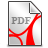# enabling a wavelet-Galerkin turbulent flow field representation with expansion coefficients in identical physical & wavelet space

When Fourier meets Navier” in a physical H(1/2) energy Hilbert space there is a global unique H(1/2) solution of the corresponding weak variation equation of the non-linear, non-stationary Navier-Stokes equations ("solution").

The standard numerical approximation method for PDE is the Ritz-Galerkin method which is usually equipped with finite element approximation spaces (FEM). In case of negative scaled Hilbert spaces the Ritz-Galerkin method accompanied by finte element approximation spaces becomes a boundary element method (BEM) related to the underlying singular equation representation.

The required finite (boundary) element approximation properties of the BEM face some challenges in case of non-linearity and/or non-periodic boundary conditions. The wavelet extension method is used to represent functions that have discontinuities and sharp peaks, and for accurately deconstructing and reconstructing finite, non-periodic and/or non-stationary signals, (MeM).Mehra M., Wavelets and Differential Equations - A short review.pdf (175.43KB)Mehra M., Wavelets and Differential Equations - A short review.pdf (175.43KB)

Here we go with a collection of some useful supporting tool sets:Braun K., Generalized wavelet theory and non-linear, non-periodic boundary value problems_1.pdf (439.35KB)Braun K., Generalized wavelet theory and non-linear, non-periodic boundary value problems_1.pdf (439.35KB)